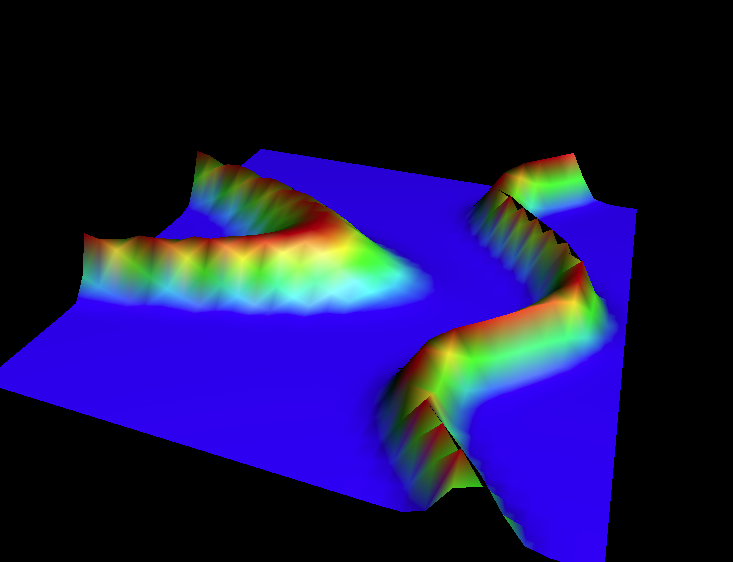# Geometric Analysis

#### MWF 1:50 -- 2:40 A-304 Wells HallProfessor Thomas H Parker
A-346 Wells Hall 353-8493
parker@math.msu.edu

Office hours:
Monday: 3-4
Thursday 2-3
Friday: 2:45-3:45
and by appointment (email to set up time).

Goals: This course is an introduction to geometric analysis on Riemannian manifolds. It introduces some of the important geometric PDEs on manifolds, including examples of linear and non-linear elliptic equations and linear and non-linear parabolic (i.e. heat flow) equations. The emphasis is on quickly acquiring a working knowledge of the tools and ideas of the subject.

Prerequisites: Familiarity with manifolds (vector fields, differential forms, tangent and tensor bundles). It will be very useful, but not strictly necessary, to have an understanding of Hilbert and Banach spaces (e.g. Chapters 3-5 of Rudin's ``Real and Complex Analysis'') and some knowledge of PDEs.

Homework:   HW1    HW2    HW3    HW4

Lecture Notes:   Primer: Sections 1-4    Direct Methods

Recommended Text: Lectures on the Geometry of Manifolds by Liviu Nicolaescu.

Preliminary list of topics:

1. Connections and curvature on vector bundles.
2. A working man's introduction to elliptic theory.
3. Finding geodesics via Morse theory.
4. The Hodge Theorem and the Bochner technique.
5. Spinors and the Dirac equation.
6. The Seiberg-Witten equations.
7. The heat kernel on manifolds.
8. Time permitting: pseudo-holomorphic maps or harmonic map heat flow.